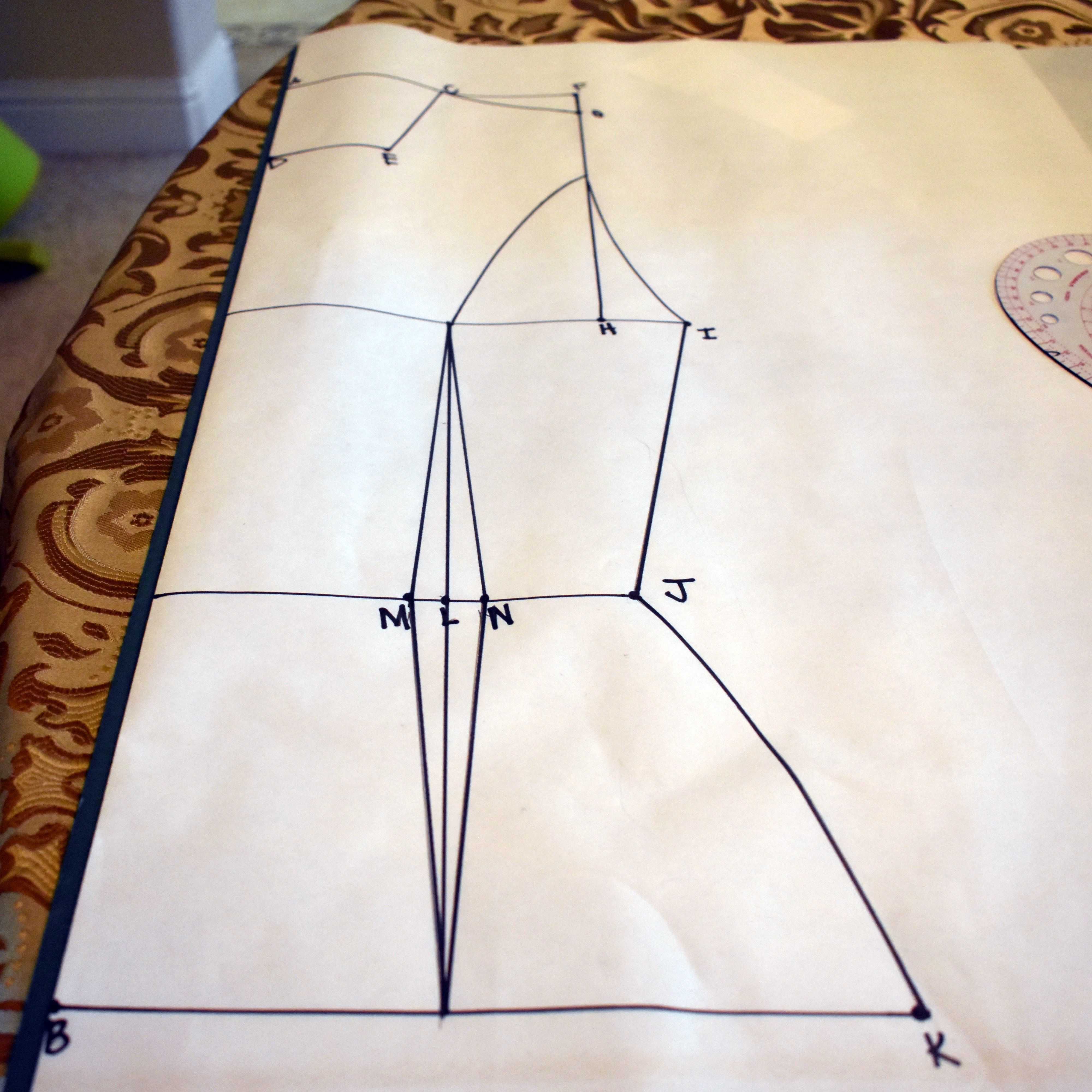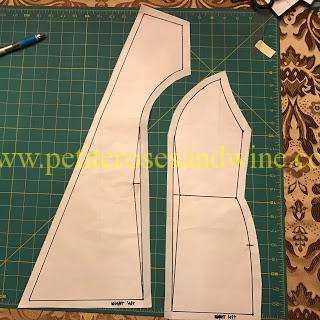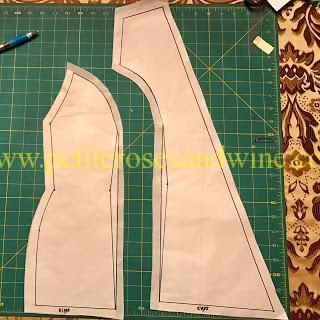# Hmong Fitted Shirt Pattern Tutorial: Bodice#### Materials::

Ruler

French Curve

Paper

Pencil

My measurements for reference:

Bust:32 inches 8.5

Waist:24 inches 6.5

Hip: 34 inches 9

Torso Length: 22 inches

#### Front of Shirt

1. Fold paper in half
2. Draw a line 5 inches from center. Mark as Point A.
3. Draw a line 22 inches down from Point A and mark as Point B. Line AB is the torso length.
4. Draw a line 3.5 inches to the right of Point A and mark as C. Line AC is the neck of the shirt. 3.5 inches should be plenty. Only adjust if the person has a much larger neck.
5. From Point C draw a diagonal line to the edge. Point D is on Line AB. Point D will be used to draw the bust line. Line CE is the wrap front part of the shirt.
6. From Point C draw a line 3.5 inches to the right. Mark as Point F. Line CF should be slightly smaller than your shoulder length.
7. Draw a line 1 inch below F and mark as Point G. Line CG should be the length of the shoulder.
8. Draw a line down 22 inches down from Point F and mark as H.
9. From Point D draw a line to the right about 10.5 inches. Mark as Point I. Line DI is the ((bust measurement +2)/4) +2. For example, my Bust Measurement is 32 inches.
• 32+2 = 34 ( I add 2 inches for room)
• 34/4= 8.5
• 8.5+2=10.5 (This allows the shirt to be able to wrap and overlap nicely)
10. The waist line is about 5 inches down from Line DI. You have 2 options to determine where to draw waistline.
• Option 1 based on your measurement. This would the length from your bust to your waist.
• Option 2 based on Line DB. For me, Line DB is 12.5 inches long. I split it so that it would be a little less than half on top and more than half on bottom. 5 inches and 7.5 inches.
11. From Line DB,  draw a line to the right about 8.5 inches and mark Point J. This line is the ((waist measurement +2)/4) +2.
12. Draw a line 11 inches to the right of Point B and mark as Point K. Line BK is the ((hip measurement +2)/4) +2.
13. Use the French Curve and ruler to connect points G&I, Points I &J, and Points J&K.
14. About 4 inches the right of Line DB on Line J mark Point L. Draw a line going through point L touching like DI and BK.
15. 0.5 inches to the right and left of Point L mark Point M and Point N.
16. Draw a diamond using Points M and N. The top point should not touch line DI. I made it about 2 inches below. This can be determined but your bust radius.
17. Use the French Curve to draw the bust seam. If the person has a big bust and large bust radius, this seam should be drawn curvier.
18. Repeat and mirror steps for the other side.#### Back of the Shirt

1. Fold the paper in half.
2. Mark Point A directly on center fold.
3. 22 inches down from Point A mark Point B. Again Line AB is the torso length.
4. Draw a line to the right of Point A 3.5 inches long and mark Point C.
5. Go down 3.5 inches from Point A and mark Pont D.
6. Draw a line 2.5 inches to the right of Point D and mark as Point E.
7. Go back to Point C and draw a line to right 3.5 inches long and mark as Point F.
8. Draw a 1-inch line down from Point F  and mark as Point G.
9. Draw a line down from Point F. I went down about 9.5 inches and marked point H. Line FH should be the same length as  Line AD from the front.
10. From the center fold,  draw a line 10. 5 inches that crosses through Point H and mark as Point I.
11. About 5 inches down from line I draw a line 8.5 inches from the center fold and mark as  Point J.
12. From Point B draw a line 11 inches to the right and mark as point K.
13. Use the French Curve and ruler to connect Points G &I, Points I&J, and Points J&K.
14. On Line J, about 4 inches from the center fold mark point L.
15. 0.5 inches to the right and left of Point L mark Point M and Point N
16. Draw a diamond. The tips of the diamond should touch Line I and Line BK. This is a waist dart.
17. Repeat and mirror steps for the other side.#### Finals Steps

1. Cut out panels and add seam allowances. The seam allowance should be about 0.5 inches.Video:

Posted in DIY